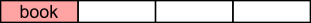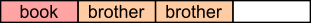#### You may also like### Consecutive Numbers

An investigation involving adding and subtracting sets of consecutive numbers. Lots to find out, lots to explore.### Calendar Capers

Choose any three by three square of dates on a calendar page...### Days and Dates

Investigate how you can work out what day of the week your birthday will be on next year, and the year after...

# Charlie's Money

##### Age 11 to 14 ShortChallenge Level

Splitting the money into parts
Charlie spent $\frac14$ of his money on a book:He then gave $\frac23$ of his remaining money to his brother:Then he had £9 left. So each of the equal parts is worth £9.

So he must have started off with 4$\times$ £9 = £36

Working backwards
After giving $\frac23$ of his money to his brother, Charlie has £9. So £9 is $\frac13$ of the amount of money Charlie had before he gave money to his brother.
So before he gave money to his brother, Charlie had £9$\times$3 = £27.

That means that after spending $\frac14$ of his money on a book, Charlie had £27. So £27 is $\frac34$ of the amount of money Charlie had before he bought the book.
So $\frac14$ of the amount of money Charlie had before he bought the book is £27$\div$3= £9.
So before he bought the book, Charlie had £9$\times$4 = £36.

Finding what fraction of his money Charlie has left
After he spent $\frac14$ of his money on a book, Charlie had $\frac34$ of his original money left.

Then he gave $\frac23$ of this $\frac34$ to his brother, leaving him with $\frac13$ of $\frac34$ of his original money.

$\frac13$ of $\frac34$ is equal to $\frac14$. So Charlie has $\frac14$ of his original money left.

So $\frac14$ of Charlie's original money is £9, so Charlie must have started off with 4$\times$ £9 = £36.

Using algebra
Let the amount of money Charlie had at the beginning be $c$.

So he spent $\frac14c$ on a book, which left him with $\frac34c$.

Then he have $\frac23$ of $\frac34c$ to his brother, leaving him with $\frac13$ of $\frac34c$, which was $£9.$

So $\frac13\times\frac34c= £9\Rightarrow\frac14c= £9\Rightarrow c= £9\times4= £36.$

You can find more short problems, arranged by curriculum topic, in our short problems collection.